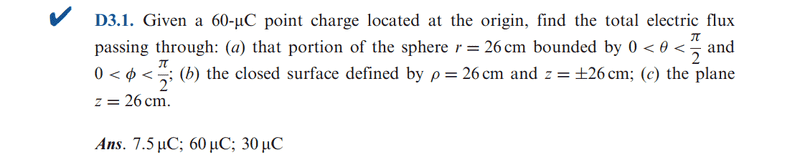# [help] finding the total electric flux

bibo_dvd
Hello Guys !

iam studing the electric flux and how the relationship between D ( electric flux density ) and E ( elecric field)

but i found this problem and i don't know which formula should i use to solve it to find the electric flux

i know that Q=(Psi)=the electric flux but i don't know how to use this to solve this problem

i tried to use the integration of the volume of the sphere which is r^2 sin(ceta)*dr*d(ceta)*d(phi)

but i didn't reach to the number in the solution ...I need your help !